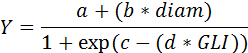SORTIE-ND
Software for spatially-explicit simulation of forest dynamics

# Logistic growth w/ size dependent asymptote

This behavior does either diameter or height growth as a function of tree size and GLI.

### Parameters for this behavior

Parameter nameDescription
Size Dep. Logistic - Diam Intercept (a)Intercept of the size dependent annual growth potential, for diameter growth.
Size Dep. Logistic - Diam Shape Param 1 (c)Shape parameter 1 for shade reduction of annual growth, for diameter growth.
Size Dep. Logistic - Diam Shape Param 2 (d)Shape parameter 2 for shade reduction of annual growth, for diameter growth.
Size Dep. Logistic - Diam Slope (b)Slope of the size dependent annual growth potential, for diameter growth.
Size Dep. Logistic - Height Intercept (a)Intercept of the size dependent annual growth potential, for height growth.
Size Dep. Logistic - Height Shape Param 1 (c)Shape parameter 1 for shade reduction of annual growth, for height growth.
Size Dep. Logistic - Height Shape Param 2 (d)Shape parameter 2 for shade reduction of annual growth, for height growth.
Size Dep. Logistic - Height Slope (b)Slope of the size dependent annual growth potential, for height growth.

### How it works

This behavior calculates annual diameter or height increases as:where

• Y = amount of diameter increase, in mm, or the amount of height increase, in cm
• a = Size Dep. Logistic - Diam Intercept (a) parameter (for diameter growth) or the Size Dep. Logistic - Height Intercept (a) parameter (for height growth)
• b = Size Dep. Logistic - Diam Slope (b) parameter (for diameter growth) or the Size Dep. Logistic - Height Slope (b) parameter (for height growth)
• c = Size Dep. Logistic - Diam Shape Param 1 (c) parameter (for diameter growth) or the Size Dep. Logistic - Height Shape Param 1 (c) parameter (for height growth)
• d = Size Dep. Logistic - Diam Shape Param 2 (d) parameter (for diameter growth) or the Size Dep. Logistic - Height Shape Param 2 (d) parameter (for height growth)
• GLI = global light index, as a percentage between 0 and 100, calculated by a light behavior
• diam = diameter (diameter at 10 cm for seedlings and saplings, DBH for adults)

For diameter growth: Assume that the number of years per timestep is X. In order to find the total amount of diameter increase for a timestep, the logistic growth equation is calculated X times, with the diameter incremented by the amount of diameter increase for the previous year. The total diameter increment is the sum of the X individual diameter increments.

For height growth: In order to find the total amount of height increase for a timestep, the behavior takes as an input the amount of diameter growth increase. Assume that the number of years per timestep is X. The amount of diameter increase is divided by X. Then the logistic growth equation is calculated X times, with the diameter incremented by the amount of diameter increase per timestep each time. The total height increment is the sum of the X individual height increments.

### How to apply it

This behavior can be applied to seedlings, saplings, and adults of any species. Any tree species/type combination to which it is applied must also have a light behavior applied. You can choose either a diam with auto height, diam only, or height only version.